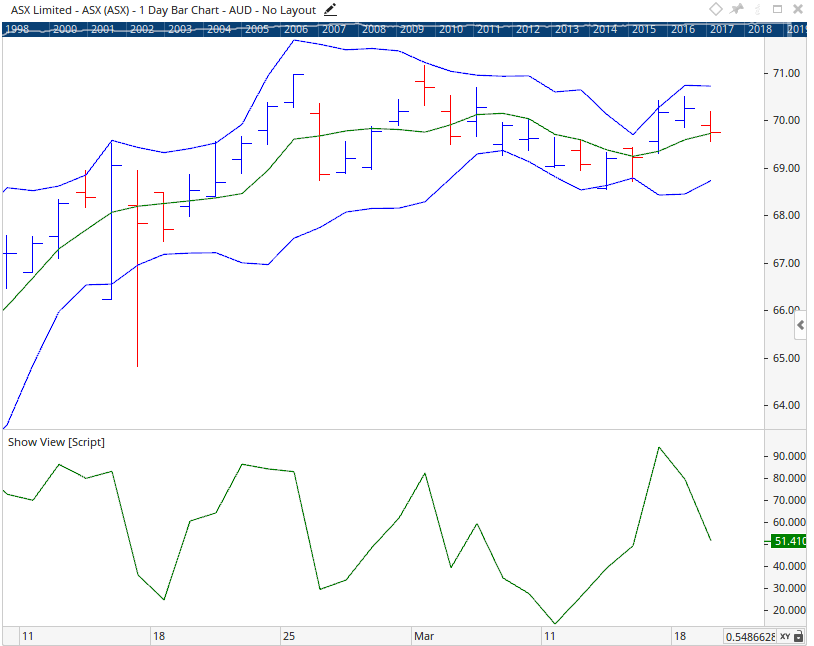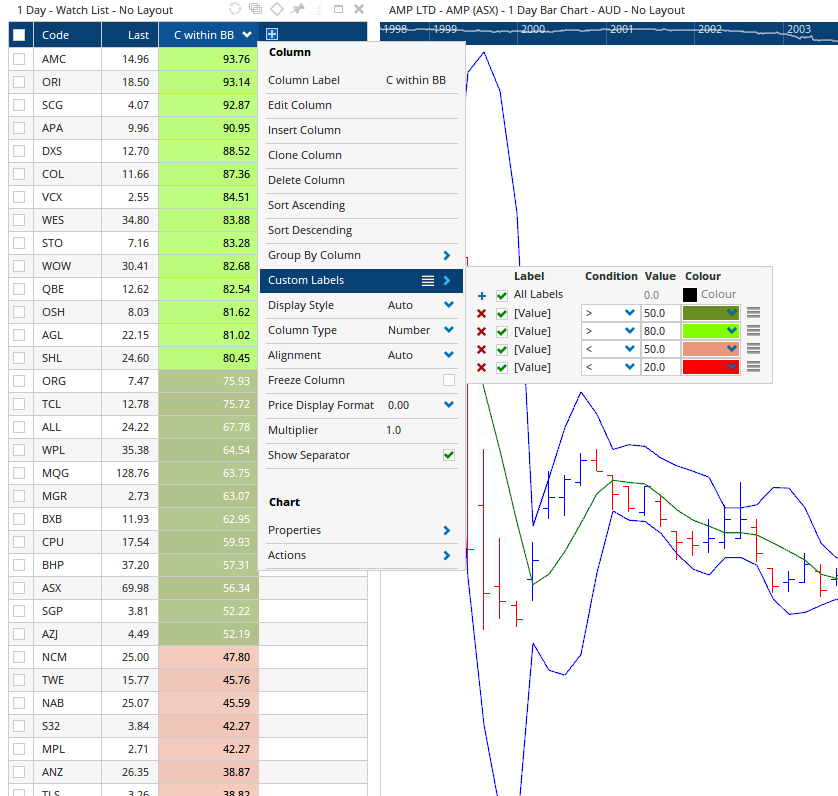Select Page

# Where does the last bar close within the bollinger bands?

Optuma Forums Optuma Scripting Where does the last bar close within the bollinger bands?

Viewing 6 posts - 1 through 6 (of 6 total)
• Author
Posts
• #51899

Hi

I’m wanting to determine where the last close falls within a set of bollinger bands. (ie is it within the lowest 20% of the range – so closer to the lower band than the upper band.)

As a first step, I’m wanting to display the last close in terms of a ratio between 0 (close is on the lower band) to 1 (close is on the upper band).  (I think I can deal with closes outside the bands later)

Anyway, here is a script to try to calculate this ratio. It starts with the distance from the close to the lower band, and divides this by the distance from the upper band to the lower band. I’m getting an error message though. Could someone suggest where I need to fix this? Thanks

Dist1 = CLOSE() – BB(DEFAULT=LowerLine, BARS=21, STYLE=Exponential);

Dist2 = BB(BARS=21, STYLE=Exponential) – BB(DEFAULT=LowerLine, BARS=21, STYLE=Exponential);

R1 = Dist1 / Dist2

#51903

Hi Steve,

The following script should return the type of result you are looking for…

This returns the position of the Close in relation to the Bollinger Bands High / Low plots as a %. A value of 0% would mean the close is the same value as the BB low plot and a value of 100% would mean the close is the same value as the BB high plot.

The end result on a Show View looks like this…#51907

Thanks Matthew – that looks good..

I do have a few odd results though so I’m trying to figure out where the problem is.

I have applied bollinger bands to a CADCHF daily chart. (bands are 21 period, geometric, applied to close)

I have also applied the following script to my chart using showview:

V1 = BB(BARS=21, STYLE=Exponential).UpperLine ;
V2 = BB(BARS=21, STYLE=Exponential).LowerLine ;

( (CLOSE() – V2) / (V1 -V2)) * 100

Image attached.

If you look at the bar of March 28, 2018, to the naked eye the close looks to be above 80% of the distance from the lower bollinger band to the upper bollinger band.

And doing the sums:

Upper band = 0.74223

Lower band = 0.72312

Close = 0.7402

Distance from lower to upper band = 0.74223-0.72312 = 0.01911

80% of this distance = 0.8 * 0.01911 = 0.015288

Add 80% of the distance from the lower bollinger band to the lower bollinger band = 0.7402 + 0.015288 = 0.738408

As the close (0.7402) is higher than 80% of the distance from the lower to the upper bollinger band (0.738408), the script should return a result of > 80. As you can see from the horizontal line set at 80, the script returns a result of < 80.

Hoping somebody can see what it is that I’m missing.

Regards
Steve

#51915

Hi Steve,

You mention the Bollinger bands applied to the chart are using a Calc Style of Geometric, however the script is using a Calc style of Exponential, so there’s a mismatch between the script and the chart.

This may explain the variations you are seeing in the results.

#51919

Yes! Thank you Matthew

#51923

Hi Steve,

One thing i thought i’d mention is that this script would work well in a Watchlist, combined with rules for colouring.  Using these rules you can easily identify codes that are within a specific range (top 20%, bottom 20%, etc).

Below is a screen shot example of what i mean…